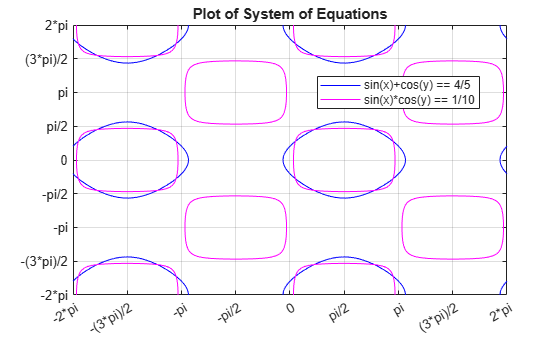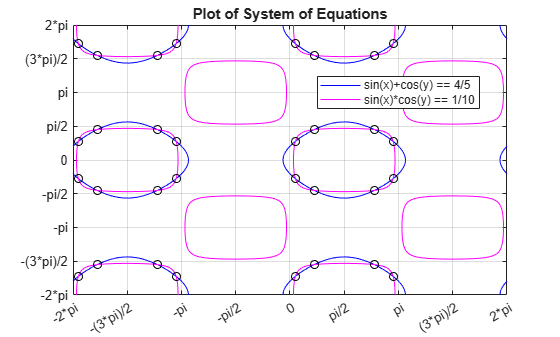# Solve System of Algebraic Equations

This topic shows you how to solve a system of equations symbolically using Symbolic Math Toolbox™. This toolbox offers both numeric and symbolic equation solvers. For a comparison of numeric and symbolic solvers, see Select Numeric or Symbolic Solver.

### Handle the Output of `solve`

Suppose you have the system

`$\begin{array}{l}{\mathit{x}}^{2}{\mathit{y}}^{2}=0\\ \mathit{x}-\frac{\mathit{y}}{2}=\alpha \text{\hspace{0.17em}},\end{array}$`

and you want to solve for $x$ and $y$. First, create the necessary symbolic objects.

`syms x y a`

There are several ways to address the output of `solve`. One way is to use a two-output call. The call returns the following.

`[solx,soly] = solve(x^2*y^2 == 0, x-y/2 == a)`
```solx =  $\left(\begin{array}{c}a\\ 0\end{array}\right)$```
```soly =  $\left(\begin{array}{c}0\\ -2 a\end{array}\right)$```

Modify the first equation to ${x}^{2}{y}^{2}=1$. The new system has more solutions. Four distinct solutions are produced.

`[solx,soly] = solve(x^2*y^2 == 1, x-y/2 == a)`
```solx =  $\left(\begin{array}{c}\frac{a}{2}-\frac{\sqrt{{a}^{2}-2}}{2}\\ \frac{a}{2}-\frac{\sqrt{{a}^{2}+2}}{2}\\ \frac{a}{2}+\frac{\sqrt{{a}^{2}-2}}{2}\\ \frac{a}{2}+\frac{\sqrt{{a}^{2}+2}}{2}\end{array}\right)$```
```soly =  $\left(\begin{array}{c}-a-\sqrt{{a}^{2}-2}\\ -a-\sqrt{{a}^{2}+2}\\ \sqrt{{a}^{2}-2}-a\\ \sqrt{{a}^{2}+2}-a\end{array}\right)$```

Since you did not specify the dependent variables, `solve` uses `symvar` to determine the variables.

This way of assigning output from `solve` is quite successful for “small” systems. For instance, if you have a 10-by-10 system of equations, typing the following is both awkward and time consuming.

```[x1,x2,x3,x4,x5,x6,x7,x8,x9,x10] = solve(...) ```

To circumvent this difficulty, `solve` can return a structure whose fields are the solutions. For example, solve the system of equations `u^2 - v^2 = a^2`, `u + v = 1`, `a^2 - 2*a = 3`. The solver returns its results enclosed in a structure.

```syms u v a S = solve(u^2 - v^2 == a^2, u + v == 1, a^2 - 2*a == 3)```
```S = struct with fields: a: [2x1 sym] u: [2x1 sym] v: [2x1 sym] ```

The solutions for `a` reside in the “`a`-field” of `S`.

`S.a`
```ans =  $\left(\begin{array}{c}-1\\ 3\end{array}\right)$```

Similar comments apply to the solutions for `u` and `v`. The structure `S` can now be manipulated by the field and index to access a particular portion of the solution. For example, to examine the second solution, you can use the following statement to extract the second component of each field.

`s2 = [S.a(2),S.u(2),S.v(2)]`
`s2 = $\left(\begin{array}{ccc}3& 5& -4\end{array}\right)$`

The following statement creates the solution matrix `M` whose rows comprise the distinct solutions of the system.

`M = [S.a,S.u,S.v]`
```M =  $\left(\begin{array}{ccc}-1& 1& 0\\ 3& 5& -4\end{array}\right)$```

Clear `solx` and `soly` for further use.

`clear solx soly`

### Solve a Linear System of Equations

Linear systems of equations can also be solved using matrix division. For example, solve this system.

```clear u v x y syms u v x y eqns = [x + 2*y == u, 4*x + 5*y == v]; S = solve(eqns); sol = [S.x;S.y]```
```sol =  $\left(\begin{array}{c}\frac{2 v}{3}-\frac{5 u}{3}\\ \frac{4 u}{3}-\frac{v}{3}\end{array}\right)$```
```[A,b] = equationsToMatrix(eqns,x,y); z = A\b```
```z =  $\left(\begin{array}{c}\frac{2 v}{3}-\frac{5 u}{3}\\ \frac{4 u}{3}-\frac{v}{3}\end{array}\right)$```

Thus, `sol` and `z` produce the same solution, although the results are assigned to different variables.

### Return the Full Solution of a System of Equations

`solve` does not automatically return all solutions of an equation. To return all solutions along with the parameters in the solution and the conditions on the solution, set the `ReturnConditions` option to `true`.

Consider the following system of equations:

`$\begin{array}{l}\mathrm{sin}\left(\mathit{x}\right)+\mathrm{cos}\left(\mathit{y}\right)=\frac{4}{5}\\ \mathrm{sin}\left(\mathit{x}\right)\text{\hspace{0.17em}}\mathrm{cos}\left(\mathit{y}\right)=\frac{1}{10}\end{array}$`

Visualize the system of equations using `fimplicit`. To set the x-axis and y-axis values in terms of `pi`, get the axes handles using `axes` in `a`. Create the symbolic array `S` of the values `-2*pi` to `2*pi` at intervals of `pi/2`. To set the ticks to `S`, use the `XTick` and `YTick` properties of `a`. To set the labels for the x-and y-axes, convert `S` to character vectors. Use `arrayfun` to apply `char` to every element of `S` to return `T`. Set the `XTickLabel` and `YTickLabel` properties of `a` to `T`.

```syms x y eqn1 = sin(x)+cos(y) == 4/5; eqn2 = sin(x)*cos(y) == 1/10; a = axes; fimplicit(eqn1,[-2*pi 2*pi],'b'); hold on grid on fimplicit(eqn2,[-2*pi 2*pi],'m'); L = sym(-2*pi:pi/2:2*pi); a.XTick = double(L); a.YTick = double(L); M = arrayfun(@char, L, 'UniformOutput', false); a.XTickLabel = M; a.YTickLabel = M; title('Plot of System of Equations') legend('sin(x)+cos(y) == 4/5','sin(x)*cos(y) == 1/10',... 'Location','best','AutoUpdate','off')```The solutions lie at the intersection of the two plots. This shows the system has repeated, periodic solutions. To solve this system of equations for the full solution set, use `solve` and set the `ReturnConditions` option to `true`.

`S = solve(eqn1,eqn2,'ReturnConditions',true)`
```S = struct with fields: x: [2x1 sym] y: [2x1 sym] parameters: [z z1] conditions: [2x1 sym] ```

`solve` returns a structure `S` with the fields `S.x` for the solution to `x`, `S.y` for the solution to `y`, `S.parameters` for the parameters in the solution, and `S.conditions` for the conditions on the solution. Elements of the same index in `S.x`, `S.y`, and `S.conditions` form a solution. Thus, `S.x(1)`, `S.y(1)`, and `S.conditions(1)` form one solution to the system of equations. The parameters in `S.parameters` can appear in all solutions.

Index into `S` to return the solutions, parameters, and conditions.

`S.x`
```ans =  $\left(\begin{array}{c}{z}_{1}\\ {z}_{1}\end{array}\right)$```
`S.y`
```ans =  $\left(\begin{array}{c}z\\ z\end{array}\right)$```
`S.parameters`
`ans = $\left(\begin{array}{cc}z& {z}_{1}\end{array}\right)$`
`S.conditions`
```ans =  ```

### Solve a System of Equations Under Conditions

To solve the system of equations under conditions, specify the conditions in the input to `solve`.

Solve the system of equations considered above for `x` and `y` in the interval `-2*pi` to `2*pi`. Overlay the solutions on the plot using `scatter`.

```Srange = solve(eqn1, eqn2, -2*pi < x, x < 2*pi, -2*pi < y, y < 2*pi, 'ReturnConditions', true); scatter(Srange.x,Srange.y,'k')```### Work with Solutions, Parameters, and Conditions Returned by `solve`

You can use the solutions, parameters, and conditions returned by `solve` to find solutions within an interval or under additional conditions. This section has the same goal as the previous section, to solve the system of equations within a search range, but with a different approach. Instead of placing conditions directly, it shows how to work with the parameters and conditions returned by `solve`.

For the full solution `S` of the system of equations, find values of `x` and `y` in the interval `-2*pi` to `2*pi` by solving the solutions `S.x` and `S.y` for the parameters `S.parameters` within that interval under the condition `S.conditions`.

Before solving for `x` and `y` in the interval, assume the conditions in `S.conditions` using `assume` so that the solutions returned satisfy the condition. Assume the conditions for the first solution.

`assume(S.conditions(1))`

Find the parameters in `S.x` and `S.y`.

`paramx = intersect(symvar(S.x),S.parameters)`
`paramx = ${z}_{1}$`
`paramy = intersect(symvar(S.y),S.parameters)`
`paramy = $z$`

Solve the first solution of `x` for the parameter `paramx`.

`solparamx(1,:) = solve(S.x(1) > -2*pi, S.x(1) < 2*pi, paramx)`
```solparamx =  $\left(\begin{array}{cccc}\pi +\mathrm{asin}\left(\frac{\sqrt{6}}{10}-\frac{2}{5}\right)& \mathrm{asin}\left(\frac{\sqrt{6}}{10}-\frac{2}{5}\right)-\pi & -\mathrm{asin}\left(\frac{\sqrt{6}}{10}-\frac{2}{5}\right)& -2 \pi -\mathrm{asin}\left(\frac{\sqrt{6}}{10}-\frac{2}{5}\right)\end{array}\right)$```

Similarly, solve the first solution of `y` for `paramy`.

`solparamy(1,:) = solve(S.y(1) > -2*pi, S.y(1) < 2*pi, paramy)`
```solparamy =  $\left(\begin{array}{cccc}\mathrm{acos}\left(\frac{\sqrt{6}}{10}+\frac{2}{5}\right)& \mathrm{acos}\left(\frac{\sqrt{6}}{10}+\frac{2}{5}\right)-2 \pi & -\mathrm{acos}\left(\frac{\sqrt{6}}{10}+\frac{2}{5}\right)& 2 \pi -\mathrm{acos}\left(\frac{\sqrt{6}}{10}+\frac{2}{5}\right)\end{array}\right)$```

Clear the assumptions set by `S.conditions(1)` using `assume`. Call `asumptions` to check that the assumptions are cleared.

```assume(S.parameters,'clear') assumptions```
``` ans = Empty sym: 1-by-0 ```

Assume the conditions for the second solution.

`assume(S.conditions(2))`

Solve the second solution to `x` and `y` for the parameters `paramx` and `paramy`.

`solparamx(2,:) = solve(S.x(2) > -2*pi, S.x(2) < 2*pi, paramx)`
```solparamx =  ```
`solparamy(2,:) = solve(S.y(2) > -2*pi, S.y(2) < 2*pi, paramy)`
```solparamy =  ```

The first rows of `paramx` and `paramy` form the first solution to the system of equations, and the second rows form the second solution.

To find the values of `x` and `y` for these values of `paramx` and `paramy`, use `subs` to substitute for `paramx` and `paramy` in `S.x` and `S.y`.

```solx(1,:) = subs(S.x(1), paramx, solparamx(1,:)); solx(2,:) = subs(S.x(2), paramx, solparamx(2,:))```
```solx =  ```
```soly(1,:) = subs(S.y(1), paramy, solparamy(1,:)); soly(2,:) = subs(S.y(2), paramy, solparamy(2,:))```
```soly =  ```

Note that `solx` and `soly` are the two sets of solutions to `x` and to `y`. The full sets of solutions to the system of equations are the two sets of points formed by all possible combinations of the values in `solx` and `soly`.

Plot these two sets of points using `scatter`. Overlay them on the plot of the equations. As expected, the solutions appear at the intersection of the plots of the two equations.

```for i = 1:length(solx(1,:)) for j = 1:length(soly(1,:)) scatter(solx(1,i), soly(1,j), 'k') scatter(solx(2,i), soly(2,j), 'k') end end```### Convert Symbolic Results to Numeric Values

Symbolic calculations provide exact accuracy, while numeric calculations are approximations. Despite this loss of accuracy, you might need to convert symbolic results to numeric approximations for use in numeric calculations. For a high-accuracy conversion, use variable-precision arithmetic provided by the `vpa` function. For standard accuracy and better performance, convert to double precision using `double`.

Use `vpa` to convert the symbolic solutions `solx` and `soly` to numeric form.

`vpa(solx)`
```ans =  $\left(\begin{array}{cccc}2.9859135500977407388300518406219& -3.2972717570818457380952349259371& 0.15567910349205249963259154265761& -6.1275062036875339772926952239014\\ 0.70095651347102524787213653614929& 2.4406361401187679905905068471302& -5.5822287937085612290531502304097& -3.8425491670608184863347799194288\end{array}\right)$```
`vpa(soly)`
```ans =  $\left(\begin{array}{cccc}0.86983981332387137135918515549046& -5.4133454938557151055661016110685& -0.86983981332387137135918515549046& 5.4133454938557151055661016110685\\ 1.4151172233028441195987301489821& -4.8680680838767423573265566175769& -1.4151172233028441195987301489821& 4.8680680838767423573265566175769\end{array}\right)$```

### Simplify Complicated Results and Improve Performance

If results look complicated, `solve` is stuck, or if you want to improve performance, see, Troubleshoot Equation Solutions from solve Function.

## Support

#### Mathematical Modeling with Symbolic Math Toolbox

Get examples and videos